Tutorials and Lecture Notes

Tutorial on Harmonic Map with C++ source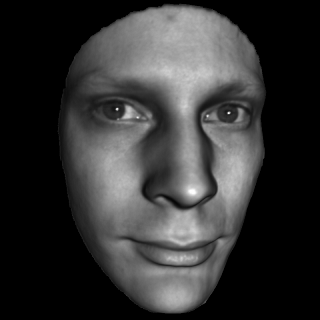This tutorial introduces the concept and the theorem of surface harmonic map, with computational algorithms. The source code and the data sets can be found here: [HTML]

Tutorial on Mesh Viewer with C++ source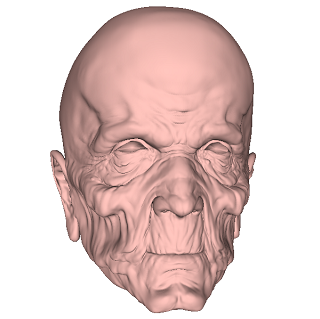This tutorial introduces a simple triangle mesh viewer using OpenGL and Glut. The source code and the data sets can be found here: [HTML]

Tutorial on Surface Ricci Flow with C++ source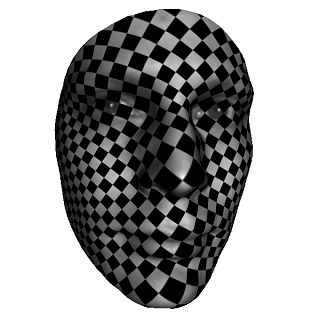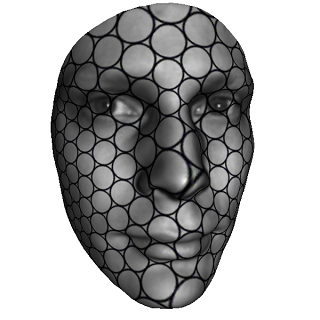This tutorial introduces the discrete surface Ricci flow method with concepts, algorithm. The source code and the data sets can be found here: [HTML]

Tutorial on Riemann with C++ source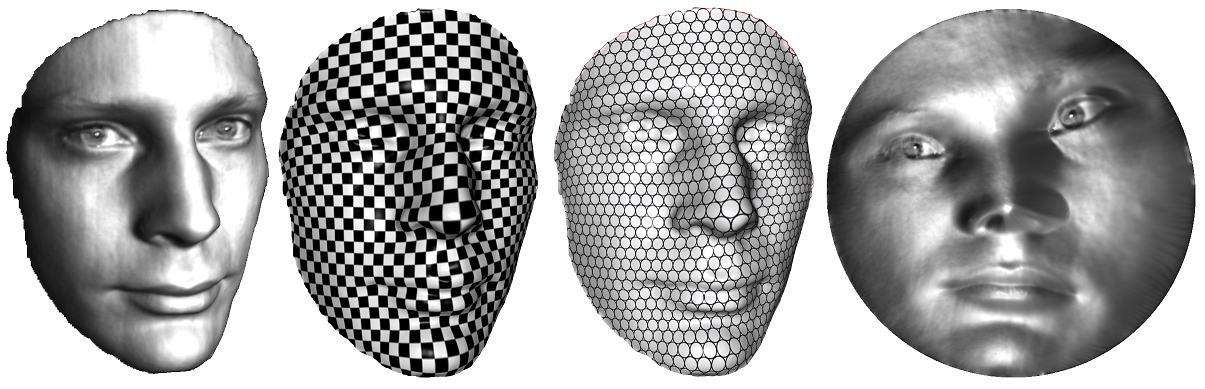This tutorial introduces the surface Riemann mapping method with concepts, algorithm. The source code and the data sets can be found here: [HTML]
Tutorial on Discrete Ricci flow

This tutorial introduces the concept of surface Ricci flow, the major theorems on the convergence of Ricci flow. Practical algorithms are explained in details, some experiemental results are demonstrated. [PDF]

Tutorial on Riemann Surface

This tutorial introduces the concept and theorems of Riemann surfaces. Practical algorithms on computing conformal structure are explained in details, some experiemental results are demonstrated.

Major algorithms inlcude spherical harmonic maps, Riemann mapping algorithm, computing harmonic 1-forms and holomorphic 1-forms etc. [PDF]

Tutorial on Surface Topology

This tutorial introduces the concept and theorems of surface topology. Practical algorithms on computing topology are explained in details, some experiemental results are demonstrated.

Major algorithms inlcude computing homology, cohomology, fundamental domain, universial covering space, shortest loops, cut graph etc. [PDF]

Lecture Notes on Computational Conformal Geometry

This is the lecture notes given in my seminar "computational conformal geometry". It covers the major concepts in topology, differential geometry, Riemann surface theories related to conformal geometry, and many major algorithms for computing conformal structures. Most directly related applications are demonstrated. [PDF]

Tutorial on Euclidean Ricci Flow

This is an early version of the tutorial introducing Euclidean Ricci flow. It includes some examples for computing flat metrics on surfaces with a single cone singularity, in other words, all curvature is concentred on a single point. [PDF]